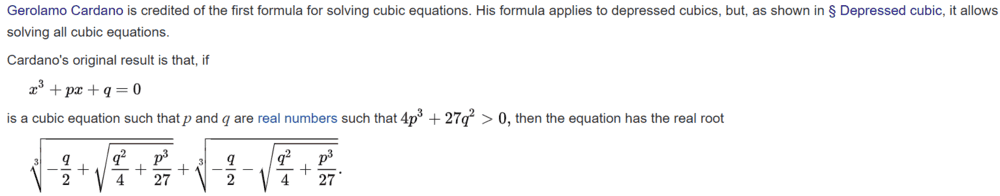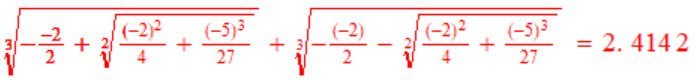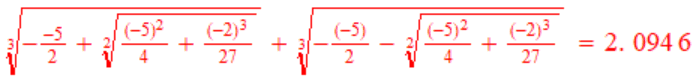# Solution of a depressed cubic equation

PainterGuy
Hi,

I was trying to solve the equation x³-2x-5=0. I solved it online and the real solution is x≈2.0946.

The following is an excerpt from the Wikipedia article on cubic equation.The cubic equation, x³-2x-5=0, is a depressed cubic and in this case 4p³+27q²>0. But the real root of equation comes out to be different, please see below.The correct real solution is x≈2.0946 therefore the value x=2.4142 is not correct. Where am I going? Could you please help me?

[Moderator's note: Moved from a technical forum and thus no template.]

Last edited by a moderator:

willem2
I think you swapped p and q.

•Delta2 and PainterGuy
PainterGuy
Thank you.

That was really silly!archaic
I'd say Prozac.

••It just needs a hug•